Test: Properties of Fluids - 2

# Test: Properties of Fluids - 2

Test Description

## 10 Questions MCQ Test Fluid Mechanics for Mechanical Engineering | Test: Properties of Fluids - 2

Test: Properties of Fluids - 2 for Mechanical Engineering 2023 is part of Fluid Mechanics for Mechanical Engineering preparation. The Test: Properties of Fluids - 2 questions and answers have been prepared according to the Mechanical Engineering exam syllabus.The Test: Properties of Fluids - 2 MCQs are made for Mechanical Engineering 2023 Exam. Find important definitions, questions, notes, meanings, examples, exercises, MCQs and online tests for Test: Properties of Fluids - 2 below.
Solutions of Test: Properties of Fluids - 2 questions in English are available as part of our Fluid Mechanics for Mechanical Engineering for Mechanical Engineering & Test: Properties of Fluids - 2 solutions in Hindi for Fluid Mechanics for Mechanical Engineering course. Download more important topics, notes, lectures and mock test series for Mechanical Engineering Exam by signing up for free. Attempt Test: Properties of Fluids - 2 | 10 questions in 30 minutes | Mock test for Mechanical Engineering preparation | Free important questions MCQ to study Fluid Mechanics for Mechanical Engineering for Mechanical Engineering Exam | Download free PDF with solutions
 1 Crore+ students have signed up on EduRev. Have you?
Test: Properties of Fluids - 2 - Question 1

### Newton’s law of viscosity for a fluid states that the shear stress is

Test: Properties of Fluids - 2 - Question 2

### A real fluid is any fluid which

Detailed Solution for Test: Properties of Fluids - 2 - Question 2

Real Fluid

• All the fluids are real as all the fluids possess viscosity.
• Real fluid is Compressible and it has some value of shear force.
• Its surface tension value in non-zero.

Ideal fluid

• Ideal fluid is Incompressible and it has zero value of shear force.
• The ideal fluid does not actually exist in nature but is sometimes used for fluid flow problems.
• An ideal fluid is a fluid that has several properties including the fact that it is:
Incompressible – the density is constant
Irrotational – the flow is smooth, no turbulence
Nonviscous – (Inviscid) fluid has no internal friction
Test: Properties of Fluids - 2 - Question 3

### When subjected to shear force, a fluid

Detailed Solution for Test: Properties of Fluids - 2 - Question 3

A fluid is a substance that deformed continuously when shear stress is applied to it irrespective of the magnitude of the shear stress. Because of the continuous deformation flow of the liquid can be seen. In other words, we can also tell that liquid can not sustain any shear load.

Test: Properties of Fluids - 2 - Question 4

An Ideal Fluid

Detailed Solution for Test: Properties of Fluids - 2 - Question 4

Ideal Fluid: A fluid, which is incompressible and is having no viscosity is known as ideai fluid. Ideal fluid is only an imaginary fluid as all the fluids, which exists have some viscosity.

Test: Properties of Fluids - 2 - Question 5

Which one of the following is defined as force per unit length?

Detailed Solution for Test: Properties of Fluids - 2 - Question 5
• Surface tension, in simple words, is defined as the force exerted on the fluid particles on a surface per unit length in the plane of the liquid surface perpendicular to either side of an imaginary line drawn to the surface.
• Mathematically, surface tension is expressed as the ratio of force to length. It is the or type of behavior of the liquid surface to contract into a minimum surface area. The standard unit of surface tension is N m-1
Test: Properties of Fluids - 2 - Question 6

Newton’s law of viscosity depends upon the

Detailed Solution for Test: Properties of Fluids - 2 - Question 6

Newton's law of viscosity:

• Viscosity is a property of fluid by virtue of which they offer resistance to shear or angular deformation.
• It is primarily due to cohesion and molecular momentum exchange between fluid layers, and as flow occurs, these effects appears as shearing stresses between the moving layers.
• According to Newton's law of viscosity, shear stress is directly proportional to the rate of angular deformation (shear strain rate) or velocity gradient across the flow.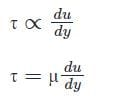where, τ = shear stress

μ = absolute or dynamic viscosity

⇒ dα/dt = rate of angular deformation (shear strain)

Test: Properties of Fluids - 2 - Question 7

The fluid which obey’s the Newton’s law of viscosity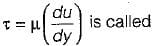Detailed Solution for Test: Properties of Fluids - 2 - Question 7

The fluid which obeys the Newton’s law of viscosity is known as Newtonion fluid e.g.: Air, water, Kerosene.

Test: Properties of Fluids - 2 - Question 8

The General relationship between shear stress (τ) and velocity Gradient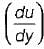for a fluid can be written as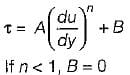Then the fluid known as

Detailed Solution for Test: Properties of Fluids - 2 - Question 8

In the expression: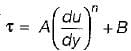If n < 1, B = 0
then it is known as pseudo plastic

Test: Properties of Fluids - 2 - Question 9

Surface tension is due to

Detailed Solution for Test: Properties of Fluids - 2 - Question 9

Surface tension a line force caused by cohesion of fluid particle at the surface, the property of cohesion enables a liquid to resist tensile stress.

Test: Properties of Fluids - 2 - Question 10

Which one of the following is correct dimension of surface tension?

Detailed Solution for Test: Properties of Fluids - 2 - Question 10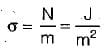## Fluid Mechanics for Mechanical Engineering

50 videos|90 docs|76 tests
 Use Code STAYHOME200 and get INR 200 additional OFF Use Coupon Code
Information about Test: Properties of Fluids - 2 Page
In this test you can find the Exam questions for Test: Properties of Fluids - 2 solved & explained in the simplest way possible. Besides giving Questions and answers for Test: Properties of Fluids - 2, EduRev gives you an ample number of Online tests for practice

## Fluid Mechanics for Mechanical Engineering

50 videos|90 docs|76 tests